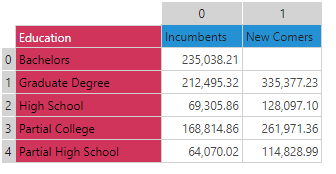#LoopVector (PQL)

Double iteratation, returning the combined result of all the looped text strings.

• Returned Output: Text
• Library: PQL \ Common \ Logical
• Version: 2020.10.000
• Compatibility:
• Can be combined with and other PQL function throughout the application.
• It CANNOT be used with MDX or VBA functions. But it can be used on MDX-based content in other parts of the application.

#### Syntax

LoopVector( <Text> , , )

* Click on the function's arguments above for more details on the input values.

• The function will iterate or loop over the supplied text and produce a concatenated text string as the result. This is useful for building dynamic lists of items.
• The supplied text can be static or any functional representation.
• The Loop iterations are a value representing the number of times the loop logic should iterate. The first is for rows. The second is for columns.
• This is a double loop - operating over a two indexes
##### Using the Loop Indexes

The loopVector function has a two internal counters called "index0" and "index1". They will resolve to positive whole numbers starting at 0 and incrementing by 1 for each loop. You can use these indexes to drive logic in your formulas as you dynamically create text.

Also see Loop

#### Examples

This example uses the following grid:Notice that "index0" and "index1" are used to automatically change the row and column positions in the data function. They start at 0 and changes 2 times for rows and 2 times for columns. Further, the data function starts by adding a 1 to index0, so that the first row computed is "1". The cells then computed are (1,0); (1,1); (2,0); (2,1)

LoopVector (Bullet("The value is " + data(index0 +1,index1)) , 2, 2)

The result here is of this logic is below. Note that the vector loop reads the cell, then moves right. This then goes down a row and moves right again.

• The value is 212495.32
• The value is 335377.23
• The value is 69305.86
• The value is 128097.10